### 【Python】淺談梯度下降與實作(上)：為什麼是你

========================================

2. 本文中有些許高中數學，不會太硬，請放心咀嚼。

========================================

## 目次

【Python】淺談梯度下降與實作(上)：為什麼是你
【Python】淺談梯度下降與實作(中)：初階的變形者們
【Python】淺談梯度下降與實作(下)：猙獰的變形者們

## 一、為什麼是你

$f(X)=w_1X_1+w_2X_2+...+w_jX_j+b ...(1)$

$MSE=\frac{1}{n}\sum_{i=1}^{n}{(f(X^i)-Y_{gt}^i)}^2 ...(2)$

$MSE=\frac{1}{n}\sum_{i=1}^{n}{((w_1X_1^i+w_2X_2^i+...+w_jX_j^i+b)-Y_{gt}^i)}^2 ...(3)$

1. 隨機給定一批權重，約莫 10～100 組(更多也可以)，並計算每組權重的數值。
2. 以特定的技巧，如遺傳、交配、突變，來更新權重朝最小值靠攏。

$w^{j+1}=w^j-\alpha\nabla E(w)，j 為迭代次數，\alpha 為學習率。$

$\mathrm{\nabla}\ f\left(x_1,x_2\right)=\left(\frac{\partial f\left(x_1,x_2\right)}{\partial x_1},\ \frac{\partial f\left(x_1,x_2\right)}{\partial x_2}\right)=\left(2x_1+1,\ 2x_2+1\right)$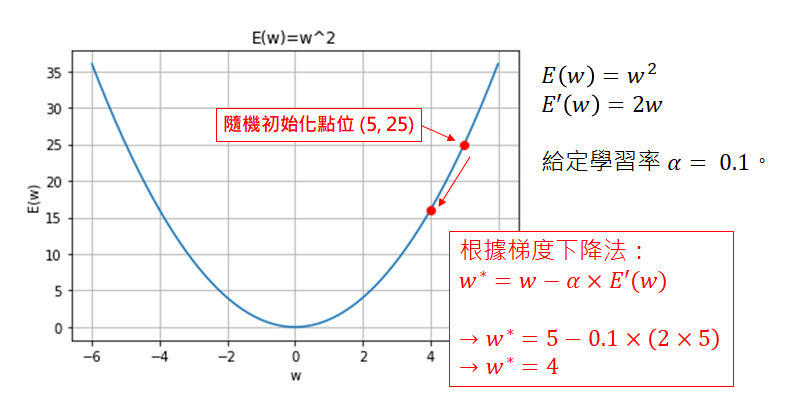1. 方向：將梯度的方向取負號，就是我們想要移動的方向。

2. 大小：由於學習率固定，因此梯度值愈大，每次移動的距離愈遠！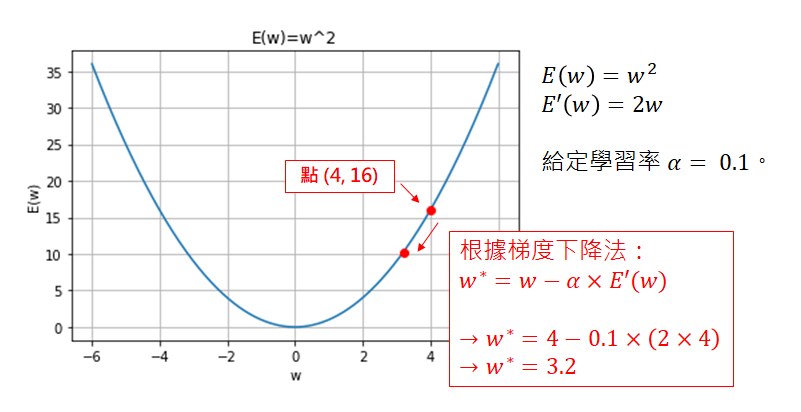1. 迭代次數達到某個值。
2. 迭代後的 loss 值間的差異小於某個數。
3. 程式執行總時間限制。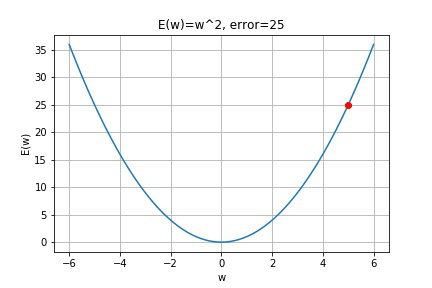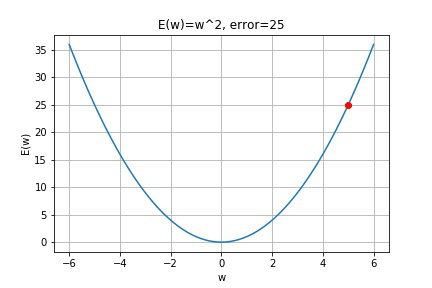# -*- coding: utf-8 -*-

import numpy as np
import matplotlib.pyplot as plt

# 學習率
alpha = 0.1

# 初始位置
w_init = 5

x = np.arange(-6, 6.1, 0.1)
y = x**2
plt.plot(x, y)

plt.xlabel('w')
plt.ylabel('E(w)')
plt.title('E(w)=w^2')
plt.grid(True)

w_old = 0; w_new = 0
for i in range(1, 11):
if i == 1:
w_old = w_init
else:
w_old = w_new

# (2*w_old) 為二次函數的的微分式
w_new = w_old - alpha * (2*w_old)

plt.plot((w_old, w_new), (w_old**2, w_new**2), 'ro-')
plt.title('E(w)=w^2, error=' + '{:.2f}'.format(abs(w_new**2)))

## 三、實戰

# -*- coding: utf-8 -*-

import numpy as np
import matplotlib.pyplot as plt

# 給定隨機種子
np.random.seed(1)

# =============================================== #
# functions

def getdata(n):
# n為產生資料量

# 給定一個固定的參數，再加上隨機變動值作為雜訊
# 其雜訊變動值介於 -10 ～ 10 之間
x = np.arange(-5, 5.1, 10/(n-1))
y = 3*x + 2 + (np.random.rand(len(x))-0.5)*20
return x, y

# =============================================== #
# 產生一組資料，共51筆。
x, y = getdata(51)

# 繪圖
plt.plot(x, y, 'bo')
plt.grid('true')
plt.ylim(-30, 30)
plt.xlabel('x')
plt.ylabel('f(x)')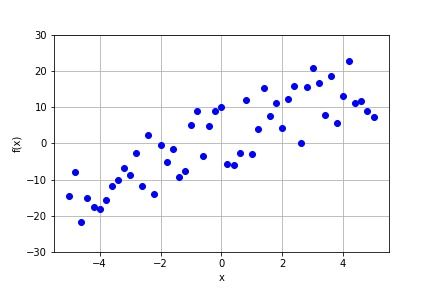$f(x)=ax+b ...(4)$

$MAE=Loss=\frac{1}{n}\sum_{i=1}^{n}{(f(x^i)-Y_t^i)}^2 ...(5)，其中n為總資料筆數， Y_t 是資料的實際值。$

$Loss(a, b)=\frac{1}{n}\sum_{i=1}^{n}{((ax^i+b)-Y_t^i)}^2$

import numpy as np
import matplotlib.pyplot as plt

# 給定隨機種子
np.random.seed(1)

# =============================================== #
# functions

def getdata(n):
# n為產生資料量

# 給定一個固定的參數，再加上隨機變動值作為雜訊
# 其雜訊變動值介於 -10 ～ 10 之間
x = np.arange(-5, 5.1, 10/(n-1))
y = 3*x + 2 + (np.random.rand(len(x))-0.5)*20
return x, y

def plot_error(x, y):
# 繪製誤差網格圖

a = np.arange(-10, 16, 1)
b = np.arange(-10, 16, 1)
mesh = np.meshgrid(a, b)

sqr_err = 0
for xs, ys in zip(x, y):
sqr_err += ((mesh*xs + mesh) - ys) ** 2
loss = sqr_err/len(x)

plt.contour(mesh, mesh, loss, 20, cmap=plt.cm.jet)
plt.xlabel('a')
plt.ylabel('b')
plt.axis('scaled')
plt.title('function loss')
plt.colorbar()

# =============================================== #
# 產生一組資料
x, y = getdata(51)
plot_error(x, y)$w^{j+1}=w^j-\alpha\nabla E(w)，j 為迭代次數，\alpha 為學習率。$

$$\mathrm{\nabla} Loss\left(a,b\right)=\left(\frac{\partial Loss\left(a,b\right)}{\partial a},\ \frac{\partial Loss\left(a,b\right)}{\partial b}\right)$$

$$\frac{\partial\ Loss\left(a,\ b\right)}{\partial a}=\frac{2}{n}\sum_{i=1}^{n}{\left(ax^i+b-Y_t^i\right)\times\left(x^i\right)}$$

$$\frac{\partial\ Loss\left(a,\ b\right)}{\partial b}=\frac{2}{n}\sum_{i=1}^{n}{\left(ax^i+b-Y_t^i\right)\times\left(1\right)}$$

def gradient(a, b, x, y):
grad_a = 2 * np.mean((a*x + b - y) * (x))
grad_b = 2 * np.mean((a*x + b - y) * (1))
return grad_a, grad_b

def update(a, b, grad_a, grad_b, alpha):
a_new = a - alpha * grad_a
b_new = b - alpha * grad_b
return a_new, b_new

def mse(a, b, x, y):
sqr_err = ((a*x + b) - y) ** 2
loss = np.mean(sqr_err)
return loss

class my_BGD:
def __init__(self, a, b, x, y, alpha):
self.a = a
self.b = b
self.x = x
self.y = y
self.alpha = alpha

self.a_old = a
self.b_old = b

self.loss = None;

# Loss function
def mse(self):
sqr_err = ((self.a*self.x + self.b) - self.y) ** 2
return np.mean(sqr_err)

grad_a = 2 * np.mean((self.a*self.x + self.b - self.y) * (self.x))
grad_b = 2 * np.mean((self.a*self.x + self.b - self.y) * (1))

def update(self):
# 計算梯度

# 梯度更新
self.a_old = self.a
self.b_old = self.b
self.a = self.a - self.alpha * grad_a
self.b = self.b - self.alpha * grad_b
self.loss = self.mse();

# 初始設定
alpha = 0.1

# 從 -9 開始，能看得更明顯
a = -9; b = -9

# 類別 my_BGD 初始化
mlclass = my_BGD(a, b, x, y, alpha)

# 畫張圖看一下
yp = a*x + b
h, = plt.plot(x, yp, 'r-')
plt.title('Initial, loss='+'{:.2f}'.format(mlclass.mse()))

# 開始迭代 10 次
for i in range(1, 11):
mlclass.update()
print('iter='+str(i)+', loss='+'{:.2f}'.format(mlclass.loss))

# 再畫張圖來看看
yp = mlclass.a*x + mlclass.b

h.remove()
h, = plt.plot(x, yp, 'r-')
plt.title('iter:'+str(i)+', loss='+'{:.2f}'.format(mlclass.loss))# 初始設定
alpha = 0.1

# 從 -9 開始，能看得更明顯
a = -9; b = -9

# 初始化
mlclass = my_BGD(a, b, x, y, alpha)

plt.plot(a, b, 'ro-')
plt.title('Initial, loss='+'{:.2f}'.format(mlclass.mse())+'\na='+
'{:.2f}'.format(a)+', b='+'{:.2f}'.format(b))

# 開始迭代
for i in range(1, 11):
mlclass.update()
print('iter='+str(i)+', loss='+'{:.2f}'.format(mlclass.loss))

plt.plot((mlclass.a_old, mlclass.a), (mlclass.b_old, mlclass.b), 'ro-')
plt.title('iter='+str(i)+', loss='+'{:.2f}'.format(mlclass.loss)+'\na='+
'{:.2f}'.format(mlclass.a)+', b='+'{:.2f}'.format(mlclass.b))1. 當資料量過大的時候難以迭代，因為記憶體會炸裂。
2. 當愈到梯度消失的高原區，模型會找不到收斂的方向而停下來。
3. 當誤差空間非常崎嶇時，難以給定學習率。

例如下圖是 CIFAR-10 dataset 使用 VGG-56 訓練的誤差空間：
在這種情況下，太大的學習率就容易從山谷跳上高原；太小則是被困在區域最小值。圖片來源：VISUALIZING THE LOSS LANDSCAPE OF NEURAL NETS

【Python】淺談梯度下降與實作(中)：初階的變形者們

## 附錄

1. 完整的程式碼，誤差空間的收斂過程。

# -*- coding: utf-8 -*-
# 說明：誤差空間的收斂過程
#
# Shayne, 2019.09.10

import numpy as np
import matplotlib.pyplot as plt

# 給定隨機種子，使每次執行結果保持一致
np.random.seed(1)

def getdata(n):
# n為產生資料量
x = np.arange(-5, 5.1, 10/(n-1))
# 給定一個固定的參數，再加上隨機變動值作為雜訊，其變動值介於 +-10 之間
y = 3*x + 2 + (np.random.rand(len(x))-0.5)*20
return x, y

def plot_error(x, y):
a = np.arange(-10, 16, 1)
b = np.arange(-10, 16, 1)
mesh = np.meshgrid(a, b)

sqr_err = 0
for xs, ys in zip(x, y):
sqr_err += ((mesh*xs + mesh) - ys) ** 2
loss = sqr_err/len(x)

plt.contour(mesh, mesh, loss, 20, cmap=plt.cm.jet)
plt.xlabel('a')
plt.ylabel('b')
plt.axis('scaled')
plt.title('function loss')

class my_BGD:
def __init__(self, a, b, x, y, alpha):
self.a = a
self.b = b
self.x = x
self.y = y
self.alpha = alpha

self.a_old = a
self.b_old = b

self.loss = self.mse();

# Loss function
def mse(self):
sqr_err = ((self.a*self.x + self.b) - self.y) ** 2
return np.mean(sqr_err)

grad_a = 2 * np.mean((self.a*self.x + self.b - self.y) * (self.x))
grad_b = 2 * np.mean((self.a*self.x + self.b - self.y) * (1))

def update(self):
# 計算梯度

# 梯度更新
self.a_old = self.a
self.b_old = self.b
self.a = self.a - self.alpha * grad_a
self.b = self.b - self.alpha * grad_b
self.loss = self.mse();

# =============================================== #
# 隨機產生一組資料
x, y = getdata(51)

# 繪製誤差空間底圖
plot_error(x, y)

# =============================================== #

# 初始設定
alpha = 0.1

# 從 -9 開始，能看得更明顯
a = -9; b = -9

# 初始化
mlclass = my_BGD(a, b, x, y, alpha)

plt.plot(a, b, 'ro-')
plt.title('Initial, loss='+'{:.2f}'.format(mlclass.loss)+'\na='+
'{:.2f}'.format(a)+', b='+'{:.2f}'.format(b))

# 開始迭代
for i in range(1, 11):
mlclass.update()
print('iter='+str(i)+', loss='+'{:.2f}'.format(mlclass.loss))

plt.plot((mlclass.a_old, mlclass.a), (mlclass.b_old, mlclass.b), 'ro-')
plt.title('iter='+str(i)+', loss='+'{:.2f}'.format(mlclass.loss)+'\na='+
'{:.2f}'.format(mlclass.a)+', b='+'{:.2f}'.format(mlclass.b))

2. 製作 GIF 的小程式，本文的動圖是在每次迭代中存檔，最後再批次轉成 GIF 檔

# -*- coding: utf-8 -*-
# 製作 GIF

import os
import imageio

images = []
img_dir = 'path/to/your/folder'

for dirPath, _, fileNames in os.walk(img_dir):
fileNames.sort(key=lambda x:int(x[:-4]))
for name in fileNames:
file_path = os.path.join(dirPath, name)
imageio.mimsave('path/to/your/filename.gif', images, duration=0.5)